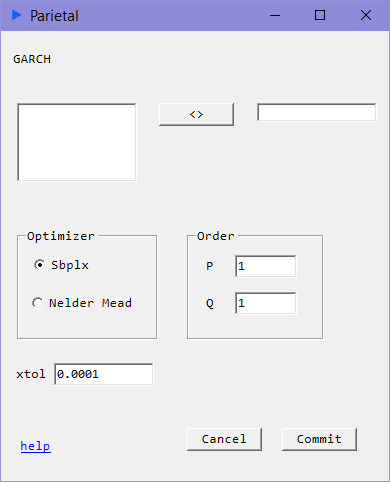# GARCH

## Time Series Volatility#### Description#

Consider the following.

• Autocorrelation in squared returns
• Volatility clustering
• Conditional Normality i.e. $f(y | y_{t-1}) \sim N(0, \alpha_{0} + \alpha_{1} y^{2}_{t-1})$

The following relation would seek to model the above:

$$\sigma_{t}^{2} = \alpha_{0} + \sum_{i=1}^{q}\alpha_{i}u_{t-i}^{2} + \sum_{i=1}^{p}\beta_{i}\sigma_{t-i}^{2}$$

$p$ specifies the GARCH parameter where $p >= 0$ and $q$ specifies the ARCH parameter where $q >= 1$

GARCH(p, q) models attempt to capture volatility dynamics in time series. When $p = 0$, it is simply an ARCH(q) model. The key difference between the two is that GARCH captures long run volatility whereas ARCH models end their volatility capture at lag q.

### Inputs#

• xtol: tolerance for convergence test
• Optimizer: Sbplx, Nelder Mead

### Returns#

• coef: Coefficient estimates for $g$ for GARCH parameters and $a$ for ARCH parameters
• serr: Standard Errors calculated via Outer Product Gradient method
• tstat: t-statistics
• pval: p-values